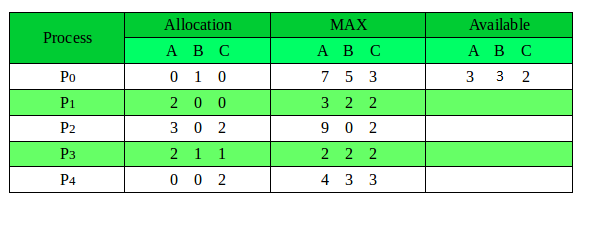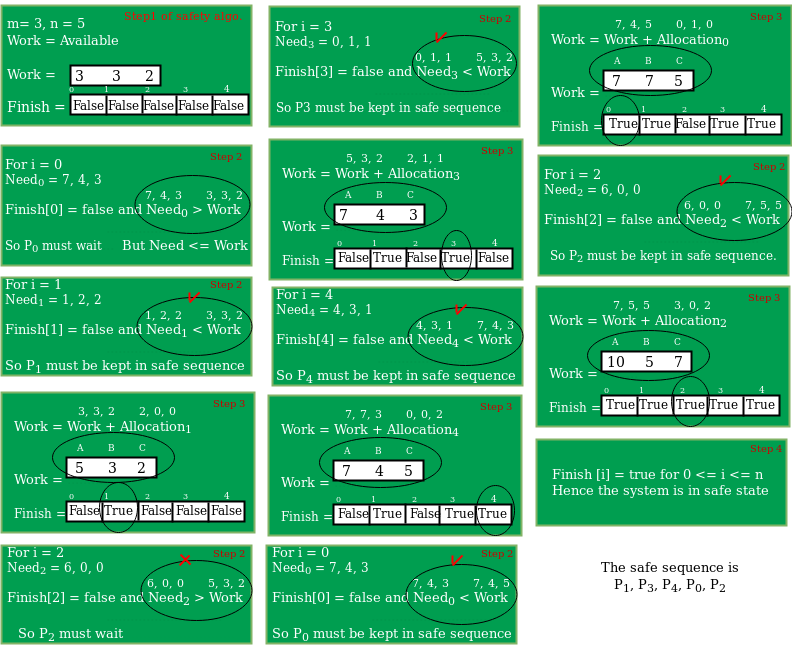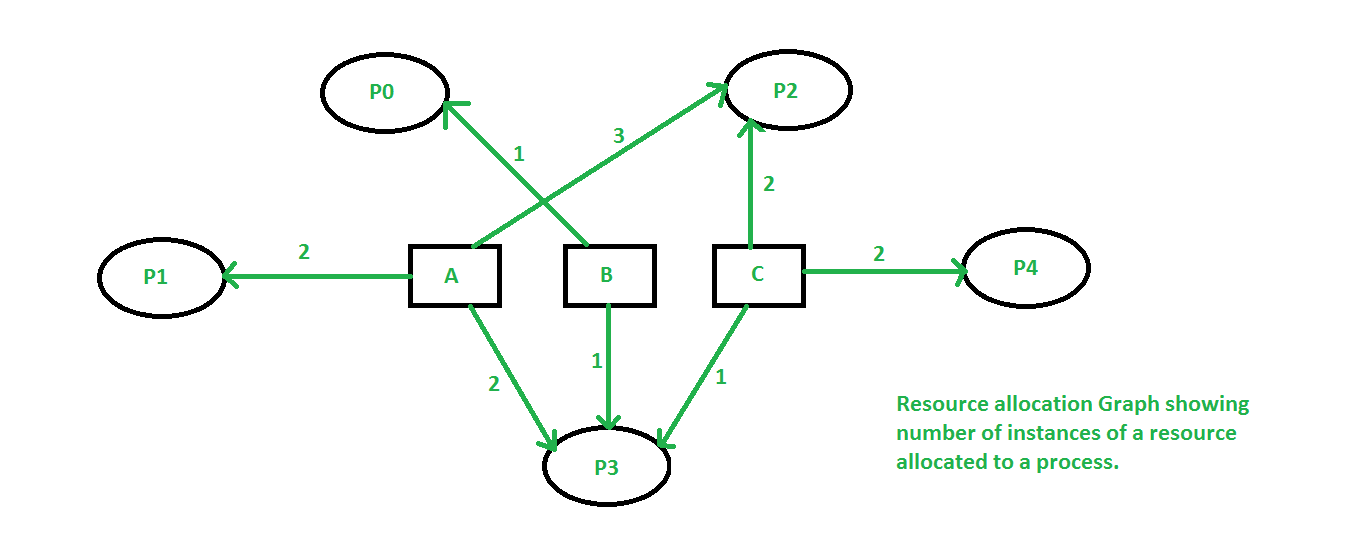Open in App
Not now

# Program for Banker’s Algorithm | Set 1 (Safety Algorithm)

• Difficulty Level : Medium
• Last Updated : 12 Jan, 2023

Prerequisite: Banker’s Algorithm The banker’s algorithm is a resource allocation and deadlock avoidance algorithm that tests for safety by simulating the allocation for predetermined maximum possible amounts of all resources, then makes an “s-state” check to test for possible activities, before deciding whether allocation should be allowed to continue. Following Data structures are used to implement the Banker’s Algorithm: Let ‘n’ be the number of processes in the system and ‘m’ be the number of resources types. Available :

• It is a 1-d array of size ‘m’ indicating the number of available resources of each type.
• Available[ j ] = k means there are ‘k’ instances of resource type Rj

Max :

• It is a 2-d array of size ‘n*m’ that defines the maximum demand of each process in a system.
• Max[ i, j ] = k means process Pi may request at most ‘k’ instances of resource type Rj.

Allocation :

• It is a 2-d array of size ‘n*m’ that defines the number of resources of each type currently allocated to each process.
• Allocation[ i, j ] = k means process Pi is currently allocated ‘k’ instances of resource type Rj

Need :

• It is a 2-d array of size ‘n*m’ that indicates the remaining resource need of each process.
• Need [ i, j ] = k means process Pi currently allocated ‘k’ instances of resource type Rj
• Need [ i, j ] = Max [ i, j ] – Allocation [ i, j ]

Allocationi specifies the resources currently allocated to process Pi and Needi specifies the additional resources that process Pi may still request to complete its task. Banker’s algorithm consist of Safety algorithm and Resource request algorithm Safety Algorithm

The algorithm for finding out whether or not a system is in a safe state can be described as follows:

1. Let Work and Finish be vectors of length ‘m’ and ‘n’ respectively. Initialize: Work= Available Finish [i]=false; for i=1,2,……,n
2. Find an i such that both a) Finish [i]=false b) Need_i<=work
if no such i exists goto step (4)
3. Work=Work + Allocation_i Finish[i]= true goto step(2)
4. If Finish[i]=true for all i, then the system is in safe state.

Safe sequence is the sequence in which the processes can be safely executed. In this post, implementation of Safety algorithm of Banker’s Algorithm is done.

## C++

 `// C++ program to illustrate Banker's Algorithm``#include``using` `namespace` `std;` `// Number of processes``const` `int` `P = 5;` `// Number of resources``const` `int` `R = 3;` `// Function to find the need of each process``void` `calculateNeed(``int` `need[P][R], ``int` `maxm[P][R],``                   ``int` `allot[P][R])``{``    ``// Calculating Need of each P``    ``for` `(``int` `i = 0 ; i < P ; i++)``        ``for` `(``int` `j = 0 ; j < R ; j++)` `            ``// Need of instance = maxm instance -``            ``//                    allocated instance``            ``need[i][j] = maxm[i][j] - allot[i][j];``}` `// Function to find the system is in safe state or not``bool` `isSafe(``int` `processes[], ``int` `avail[], ``int` `maxm[][R],``            ``int` `allot[][R])``{``    ``int` `need[P][R];` `    ``// Function to calculate need matrix``    ``calculateNeed(need, maxm, allot);` `    ``// Mark all processes as infinish``    ``bool` `finish[P] = {0};` `    ``// To store safe sequence``    ``int` `safeSeq[P];` `    ``// Make a copy of available resources``    ``int` `work[R];``    ``for` `(``int` `i = 0; i < R ; i++)``        ``work[i] = avail[i];` `    ``// While all processes are not finished``    ``// or system is not in safe state.``    ``int` `count = 0;``    ``while` `(count < P)``    ``{``        ``// Find a process which is not finish and``        ``// whose needs can be satisfied with current``        ``// work[] resources.``        ``bool` `found = ``false``;``        ``for` `(``int` `p = 0; p < P; p++)``        ``{``            ``// First check if a process is finished,``            ``// if no, go for next condition``            ``if` `(finish[p] == 0)``            ``{``                ``// Check if for all resources of``                ``// current P need is less``                ``// than work``                ``int` `j;``                ``for` `(j = 0; j < R; j++)``                    ``if` `(need[p][j] > work[j])``                        ``break``;` `                ``// If all needs of p were satisfied.``                ``if` `(j == R)``                ``{``                    ``// Add the allocated resources of``                    ``// current P to the available/work``                    ``// resources i.e.free the resources``                    ``for` `(``int` `k = 0 ; k < R ; k++)``                        ``work[k] += allot[p][k];` `                    ``// Add this process to safe sequence.``                    ``safeSeq[count++] = p;` `                    ``// Mark this p as finished``                    ``finish[p] = 1;` `                    ``found = ``true``;``                ``}``            ``}``        ``}` `        ``// If we could not find a next process in safe``        ``// sequence.``        ``if` `(found == ``false``)``        ``{``            ``cout << ``"System is not in safe state"``;``            ``return` `false``;``        ``}``    ``}` `    ``// If system is in safe state then``    ``// safe sequence will be as below``    ``cout << ``"System is in safe state.\nSafe"``         ``" sequence is: "``;``    ``for` `(``int` `i = 0; i < P ; i++)``        ``cout << safeSeq[i] << ``" "``;` `    ``return` `true``;``}` `// Driver code``int` `main()``{``    ``int` `processes[] = {0, 1, 2, 3, 4};` `    ``// Available instances of resources``    ``int` `avail[] = {3, 3, 2};` `    ``// Maximum R that can be allocated``    ``// to processes``    ``int` `maxm[][R] = {{7, 5, 3},``                     ``{3, 2, 2},``                     ``{9, 0, 2},``                     ``{2, 2, 2},``                     ``{4, 3, 3}};` `    ``// Resources allocated to processes``    ``int` `allot[][R] = {{0, 1, 0},``                      ``{2, 0, 0},``                      ``{3, 0, 2},``                      ``{2, 1, 1},``                      ``{0, 0, 2}};` `    ``// Check system is in safe state or not``    ``isSafe(processes, avail, maxm, allot);` `    ``return` `0;``}`

## Java

 `// Java program to illustrate Banker's Algorithm``import` `java.util.*;` `class` `GFG``{` `// Number of processes``static` `int` `P = ``5``;` `// Number of resources``static` `int` `R = ``3``;` `// Function to find the need of each process``static` `void` `calculateNeed(``int` `need[][], ``int` `maxm[][],``                ``int` `allot[][])``{``    ``// Calculating Need of each P``    ``for` `(``int` `i = ``0` `; i < P ; i++)``        ``for` `(``int` `j = ``0` `; j < R ; j++)` `            ``// Need of instance = maxm instance -``            ``//                 allocated instance``            ``need[i][j] = maxm[i][j] - allot[i][j];``}` `// Function to find the system is in safe state or not``static` `boolean` `isSafe(``int` `processes[], ``int` `avail[], ``int` `maxm[][],``            ``int` `allot[][])``{``    ``int` `[][]need = ``new` `int``[P][R];` `    ``// Function to calculate need matrix``    ``calculateNeed(need, maxm, allot);` `    ``// Mark all processes as infinish``    ``boolean` `[]finish = ``new` `boolean``[P];` `    ``// To store safe sequence``    ``int` `[]safeSeq = ``new` `int``[P];` `    ``// Make a copy of available resources``    ``int` `[]work = ``new` `int``[R];``    ``for` `(``int` `i = ``0``; i < R ; i++)``        ``work[i] = avail[i];` `    ``// While all processes are not finished``    ``// or system is not in safe state.``    ``int` `count = ``0``;``    ``while` `(count < P)``    ``{``        ``// Find a process which is not finish and``        ``// whose needs can be satisfied with current``        ``// work[] resources.``        ``boolean` `found = ``false``;``        ``for` `(``int` `p = ``0``; p < P; p++)``        ``{``            ``// First check if a process is finished,``            ``// if no, go for next condition``            ``if` `(finish[p] == ``false``)``            ``{``                ``// Check if for all resources of``                ``// current P need is less``                ``// than work``                ``int` `j;``                ``for` `(j = ``0``; j < R; j++)``                    ``if` `(need[p][j] > work[j])``                        ``break``;` `                ``// If all needs of p were satisfied.``                ``if` `(j == R)``                ``{``                    ``// Add the allocated resources of``                    ``// current P to the available/work``                    ``// resources i.e.free the resources``                    ``for` `(``int` `k = ``0` `; k < R ; k++)``                        ``work[k] += allot[p][k];` `                    ``// Add this process to safe sequence.``                    ``safeSeq[count++] = p;` `                    ``// Mark this p as finished``                    ``finish[p] = ``true``;` `                    ``found = ``true``;``                ``}``            ``}``        ``}` `        ``// If we could not find a next process in safe``        ``// sequence.``        ``if` `(found == ``false``)``        ``{``            ``System.out.print(``"System is not in safe state"``);``            ``return` `false``;``        ``}``    ``}` `    ``// If system is in safe state then``    ``// safe sequence will be as below``    ``System.out.print(``"System is in safe state.\nSafe"``        ``+``" sequence is: "``);``    ``for` `(``int` `i = ``0``; i < P ; i++)``        ``System.out.print(safeSeq[i] + ``" "``);` `    ``return` `true``;``}` `// Driver code``public` `static` `void` `main(String[] args)``{``    ``int` `processes[] = {``0``, ``1``, ``2``, ``3``, ``4``};` `    ``// Available instances of resources``    ``int` `avail[] = {``3``, ``3``, ``2``};` `    ``// Maximum R that can be allocated``    ``// to processes``    ``int` `maxm[][] = {{``7``, ``5``, ``3``},``                    ``{``3``, ``2``, ``2``},``                    ``{``9``, ``0``, ``2``},``                    ``{``2``, ``2``, ``2``},``                    ``{``4``, ``3``, ``3``}};` `    ``// Resources allocated to processes``    ``int` `allot[][] = {{``0``, ``1``, ``0``},``                    ``{``2``, ``0``, ``0``},``                    ``{``3``, ``0``, ``2``},``                    ``{``2``, ``1``, ``1``},``                    ``{``0``, ``0``, ``2``}};` `    ``// Check system is in safe state or not``    ``isSafe(processes, avail, maxm, allot);``}``}` `// This code has been contributed by 29AjayKumar`

## Python3

 `# Python3 program to illustrate``# Banker's Algorithm` `# Number of processes``P ``=` `5` `# Number of resources``R ``=` `3` `# Function to find the need of each process``def` `calculateNeed(need, maxm, allot):` `    ``# Calculating Need of each P``    ``for` `i ``in` `range``(P):``        ``for` `j ``in` `range``(R):``            ` `            ``# Need of instance = maxm instance -``            ``# allocated instance``            ``need[i][j] ``=` `maxm[i][j] ``-` `allot[i][j]` `# Function to find the system is in``# safe state or not``def` `isSafe(processes, avail, maxm, allot):``    ``need ``=` `[]``    ``for` `i ``in` `range``(P):``        ``l ``=` `[]``        ``for` `j ``in` `range``(R):``            ``l.append(``0``)``        ``need.append(l)``        ` `    ``# Function to calculate need matrix``    ``calculateNeed(need, maxm, allot)` `    ``# Mark all processes as infinish``    ``finish ``=` `[``0``] ``*` `P``    ` `    ``# To store safe sequence``    ``safeSeq ``=` `[``0``] ``*` `P` `    ``# Make a copy of available resources``    ``work ``=` `[``0``] ``*` `R``    ``for` `i ``in` `range``(R):``        ``work[i] ``=` `avail[i]` `    ``# While all processes are not finished``    ``# or system is not in safe state.``    ``count ``=` `0``    ``while` `(count < P):``        ` `        ``# Find a process which is not finish``        ``# and whose needs can be satisfied``        ``# with current work[] resources.``        ``found ``=` `False``        ``for` `p ``in` `range``(P):``        ` `            ``# First check if a process is finished,``            ``# if no, go for next condition``            ``if` `(finish[p] ``=``=` `0``):``            ` `                ``# Check if for all resources``                ``# of current P need is less``                ``# than work``                ``for` `j ``in` `range``(R):``                    ``if` `(need[p][j] > work[j]):``                        ``break``                    ` `                ``# If all needs of p were satisfied.``                ``if` `(j ``=``=` `R ``-` `1``):``                ` `                    ``# Add the allocated resources of``                    ``# current P to the available/work``                    ``# resources i.e.free the resources``                    ``for` `k ``in` `range``(R):``                        ``work[k] ``+``=` `allot[p][k]` `                    ``# Add this process to safe sequence.``                    ``safeSeq[count] ``=` `p``                    ``count ``+``=` `1` `                    ``# Mark this p as finished``                    ``finish[p] ``=` `1` `                    ``found ``=` `True``                ` `        ``# If we could not find a next process``        ``# in safe sequence.``        ``if` `(found ``=``=` `False``):``            ``print``(``"System is not in safe state"``)``            ``return` `False``        ` `    ``# If system is in safe state then``    ``# safe sequence will be as below``    ``print``(``"System is in safe state."``,``              ``"\nSafe sequence is: "``, end ``=` `" "``)``    ``print``(``*``safeSeq)` `    ``return` `True` `# Driver code``if` `__name__ ``=``=``"__main__"``:``    ` `    ``processes ``=` `[``0``, ``1``, ``2``, ``3``, ``4``]` `    ``# Available instances of resources``    ``avail ``=` `[``3``, ``3``, ``2``]` `    ``# Maximum R that can be allocated``    ``# to processes``    ``maxm ``=` `[[``7``, ``5``, ``3``], [``3``, ``2``, ``2``],``            ``[``9``, ``0``, ``2``], [``2``, ``2``, ``2``],``            ``[``4``, ``3``, ``3``]]` `    ``# Resources allocated to processes``    ``allot ``=` `[[``0``, ``1``, ``0``], [``2``, ``0``, ``0``],``             ``[``3``, ``0``, ``2``], [``2``, ``1``, ``1``],``             ``[``0``, ``0``, ``2``]]` `    ``# Check system is in safe state or not``    ``isSafe(processes, avail, maxm, allot)` `# This code is contributed by``# Shubham Singh(SHUBHAMSINGH10)`

## C#

 `// C# program to illustrate Banker's Algorithm``using` `System;` `class` `GFG``{``    ` `// Number of processes``static` `int` `P = 5;` `// Number of resources``static` `int` `R = 3;` `// Function to find the need of each process``static` `void` `calculateNeed(``int` `[,]need, ``int` `[,]maxm,``                ``int` `[,]allot)``{``    ``// Calculating Need of each P``    ``for` `(``int` `i = 0 ; i < P ; i++)``        ``for` `(``int` `j = 0 ; j < R ; j++)` `            ``// Need of instance = maxm instance -``            ``//             allocated instance``            ``need[i,j] = maxm[i,j] - allot[i,j];``}` `// Function to find the system is in safe state or not``static` `bool` `isSafe(``int` `[]processes, ``int` `[]avail, ``int` `[,]maxm,``            ``int` `[,]allot)``{``    ``int` `[,]need = ``new` `int``[P,R];` `    ``// Function to calculate need matrix``    ``calculateNeed(need, maxm, allot);` `    ``// Mark all processes as infinish``    ``bool` `[]finish = ``new` `bool``[P];` `    ``// To store safe sequence``    ``int` `[]safeSeq = ``new` `int``[P];` `    ``// Make a copy of available resources``    ``int` `[]work = ``new` `int``[R];``    ``for` `(``int` `i = 0; i < R ; i++)``        ``work[i] = avail[i];` `    ``// While all processes are not finished``    ``// or system is not in safe state.``    ``int` `count = 0;``    ``while` `(count < P)``    ``{``        ``// Find a process which is not finish and``        ``// whose needs can be satisfied with current``        ``// work[] resources.``        ``bool` `found = ``false``;``        ``for` `(``int` `p = 0; p < P; p++)``        ``{``            ``// First check if a process is finished,``            ``// if no, go for next condition``            ``if` `(finish[p] == ``false``)``            ``{``                ``// Check if for all resources of``                ``// current P need is less``                ``// than work``                ``int` `j;``                ``for` `(j = 0; j < R; j++)``                    ``if` `(need[p,j] > work[j])``                        ``break``;` `                ``// If all needs of p were satisfied.``                ``if` `(j == R)``                ``{``                    ``// Add the allocated resources of``                    ``// current P to the available/work``                    ``// resources i.e.free the resources``                    ``for` `(``int` `k = 0 ; k < R ; k++)``                        ``work[k] += allot[p,k];` `                    ``// Add this process to safe sequence.``                    ``safeSeq[count++] = p;` `                    ``// Mark this p as finished``                    ``finish[p] = ``true``;` `                    ``found = ``true``;``                ``}``            ``}``        ``}` `        ``// If we could not find a next process in safe``        ``// sequence.``        ``if` `(found == ``false``)``        ``{``            ``Console.Write(``"System is not in safe state"``);``            ``return` `false``;``        ``}``    ``}` `    ``// If system is in safe state then``    ``// safe sequence will be as below``        ``Console.Write(``"System is in safe state.\nSafe"``        ``+``" sequence is: "``);``    ``for` `(``int` `i = 0; i < P ; i++)``            ``Console.Write(safeSeq[i] + ``" "``);` `    ``return` `true``;``}` `// Driver code``static` `public` `void` `Main ()``{``    ``int` `[]processes = {0, 1, 2, 3, 4};` `    ``// Available instances of resources``    ``int` `[]avail = {3, 3, 2};` `    ``// Maximum R that can be allocated``    ``// to processes``    ``int` `[,]maxm = {{7, 5, 3},``                    ``{3, 2, 2},``                    ``{9, 0, 2},``                    ``{2, 2, 2},``                    ``{4, 3, 3}};` `    ``// Resources allocated to processes``    ``int` `[,]allot = {{0, 1, 0},``                    ``{2, 0, 0},``                    ``{3, 0, 2},``                    ``{2, 1, 1},``                    ``{0, 0, 2}};` `    ``// Check system is in safe state or not``    ``isSafe(processes, avail, maxm, allot);` `    ``}``}` `// This code has been contributed by ajit.`

## Javascript

 `// Number of processes``const P = 5;` `// Number of resources``const R = 3;` `// Function to find the need of each process``function` `calculateNeed(need, maxm, allot) {``  ``// Calculating Need of each P``  ``for` `(let i = 0; i < P; i++) {``    ``for` `(let j = 0; j < R; j++) {``      ``// Need of instance = maxm instance -``      ``//                    allocated instance``      ``need[i][j]=(maxm[i][j] - allot[i][j]);``    ``}``  ``}``}` `// Function to find the system is in safe state or not``function` `isSafe(processes, avail, maxm, allot) {``  ``let need = ``new` `Array(P);``  ``for``(let i=0;i work[j]) {``            ``break``;``          ``}``        ``}` `        ``// If all needs of p were satisfied.``        ``if` `(j == R) {``          ``// Add the allocated resources of``          ``// current P to the available/work``          ``// resources i.e.free the resources``          ``for` `(let k = 0; k < R; k++) {``            ``work[k] += allot[p][k];``          ``}` `          ``// Add this process to safe sequence.``          ``safeSeq[count] = p;``          ``count++;` `          ``// Mark this p as finished``          ``finish[p] = ``true``;` `          ``found = ``true``;``        ``}``      ``}``    ``}` `    ``// If we could not find a next process in safe``    ``// sequence.``    ``if` `(found == ``false``) {``      ``console.log(``"System is not in safe state"``);``      ``return` `false``;``    ``}``  ``}` `  ``// If system is in safe state then``  ``// safe sequence will be as below``  ``console.log(``"System is in safe state.\nSafe sequence is: "``);``  ``for` `(let i = 0; i < P; i++) {``    ``console.log(safeSeq[i] + ``" "``);``  ``}` `  ``return` `true``;``}` `let processes = [0, 1, 2, 3, 4];` `// Available instances of resources``let avail = [3, 3, 2];` `// Maximum R that can be allocated to processes``let maxm = [``  ``[7, 5, 3],``  ``[3, 2, 2],``  ``[9, 0, 2],``  ``[2, 2, 2],``  ``[4, 3, 3],``];` `// Resources allocated to processes``let allot = [``  ``[0, 1, 0],``  ``[2, 0, 0],``  ``[3, 0, 2],``  ``[2, 1, 1],``  ``[0, 0, 2],``];` `// Check system is in safe state or not``isSafe(processes, avail, maxm, allot);` `// 7 4 3``// 1 2 2``// 6 0 0``// 0 1 1``// 4 3 1` `// This code is contributed by ishankhandelwals.`

Output:

```System is in safe state.
Safe sequence is: 1 3 4 0 2```

Illustration : Considering a system with five processes P0 through P4 and three resources types A, B, C. Resource type A has 10 instances, B has 5 instances and type C has 7 instances. Suppose at time t0 following snapshot of the system has been taken:We must determine whether the new system state is safe. To do so, we need to execute Safety algorithm on the above given allocation chart.Following is the resource allocation graph:Executing safety algorithm shows that sequence < P1, P3, P4, P0, P2 > satisfies safety requirement. Time complexity = O(n*n*m) where n = number of processes and m = number of resources. This article is contributed by Sahil Chhabra (akku). If you like GeeksforGeeks and would like to contribute, you can also write an article using write.geeksforgeeks.org or mail your article to review-team@geeksforgeeks.org. See your article appearing on the GeeksforGeeks main page and help other Geeks. Please write comments if you find anything incorrect, or you want to share more information about the topic discussed above.

My Personal Notes arrow_drop_up